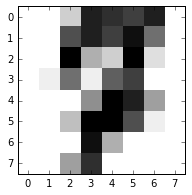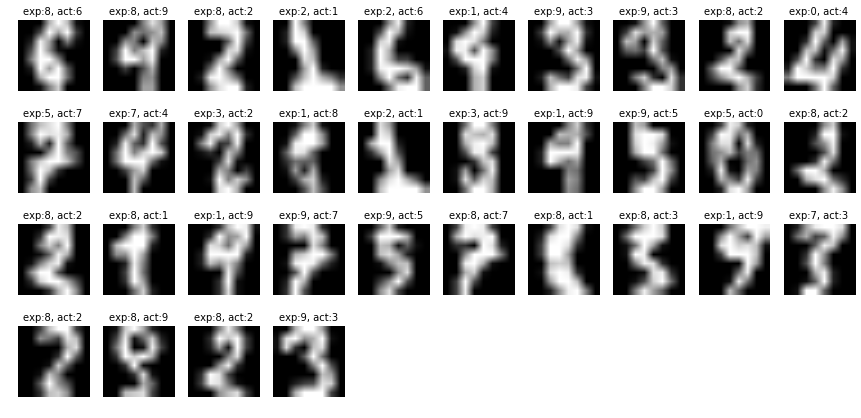# MNIST LDA Classifier

Alex Egg,

This paper will document the implementation of a handwritten digit recognition system using a Gaussian Generative Model called Linear Discrimiate Analysis. We will use the MNIST dataset which can be obtained from: http://yann.lecun.com/exdb/mnist/index.html

## MNIST Summary

MNIST has 60k training examples and 10k test examples. For this model we have 10 classes to classify: 0, 1, 2, 3, 4, 5, 6, 7, 8, 9, which represents the digits 0-9. Each example in MNIST is a 28x28 pixel image represented by 784 greyscale intensity (0-255) features. Each class will modeled by a multivariate 784-dimensional gaussian. In other words we have a 784-dimensional feature vector and 10, 768 degree multivariate gaussians.

# Iris

Before we get started on MNIST, lets work on a smaller scale w/ the iris dataset. 4 Features/target. 4 classes. So each class would have a 4D M-Gaussian. This is actually a really good model for IRIS b/c gaussians follow the random patterns in nature and iris is samples from nature.

import math
import matplotlib.pyplot as plt
%pylab inline
import numpy as np
from sklearn import datasets
from sklearn.naive_bayes import GaussianNB

gnb = GaussianNB()
gnb.fit(iris.data, iris.target)


As you can see from the Iris data, we have 150 examples, with 3 total classes. Therefore the class priori or $π_j = 1/3$

print gnb.classes_
print gnb.class_count_
print gnb.class_prior_
print gnb.sigma_ # variance of each feature per class -- not Sigma in the context of covariance matrix

[0 1 2]
[ 50.  50.  50.]
[ 0.33333333  0.33333333  0.33333333]
[[ 0.121764  0.142276  0.029504  0.011264]
[ 0.261104  0.0965    0.2164    0.038324]
[ 0.396256  0.101924  0.298496  0.073924]]


Now, what scikit did was to fit a gaussian to each of the classes (3) in the training set examples. It does that by finding the mean and the covariance from the examples. Let the Gaussian for the jth class be: $P_j = N(\mu_j, \Sigma_j)$.

$\DeclareMathOperator*{\argmax}{arg\,max}$
Then in order to classify an unknown flower, simply use the class prior w/ the class posterior (Bayes Rule) for all the classes and choose the one w/ the largest probability:

y_pred = gnb.predict(iris.data)
print("Number of mislabeled points out of a total %d points : %d" % (iris.data.shape,(iris.target != y_pred).sum()))

Number of mislabeled points out of a total 150 points : 6


Easy huh? Now, the devil is in the details: how do you calculate $P_j$, how do we estimate a gaussian for each class j?

We know that $p(\hat{x})$, the probability density function (PDF) of a multivariate Gaussian is this standard form:

Therefore the trick is to tune $\Sigma$, the covariance matrix, to model the training data.

$\Sigma$ is a p x p matrix containing all pairwise covariances, where p is the number of features in your training set:

Then, given some training points, the way to generate this matrix using numpy: np.cov(examples, rowvar=0)

Then, for each target/class (10) you will get a $\mu$ and a $\Sigma$ that you can pop into the numpy PDF routine: np.random.multivariate_normal

mean = np.array(examples.mean(0))
cov = np.cov(examples, rowvar=0)
p_x = multivariate_normal(mean=mean, cov=cov)


For iris dataset we will have 4x4 covariance matrix b/c there are 4 features in our training set., here’s an example of finding the covariance matrix $\Sigma$ on the iris trainging set. We should be able to confirm our answer

#lets split into a test and training set
from sklearn.cross_validation import train_test_split
X_train, X_test, Y_train, Y_test = train_test_split(iris.data,iris.target, test_size=0.4, random_state=4)

# lets gather all the examples from class 0
def get_examples_for_class(class_id):
examples = []
for i, example in enumerate(X_train):
if Y_train[i]==class_id:
examples.append(example)

examples = np.matrix(examples)
return examples

examples = get_examples_for_class(0)
examples.shape

(25, 4)


Now according to the above assertion this should map to a 4x4 covariance matrix. We can use numpy.cov to test this assertion and then look at how to implement the equivalent numpy.cov in python.

mean = np.array(examples.mean(0))
cov = np.cov(examples.T) # I don't know why you have to transpose the input to numpy...
print mean
print cov #should be 4x4 for iris

[ 4.964  3.416  1.44   0.24 ]
[[ 0.1049      0.06976667  0.01483333  0.00566667]
[ 0.06976667  0.10723333  0.00391667  0.00266667]
[ 0.01483333  0.00391667  0.02583333  0.00625   ]
[ 0.00566667  0.00266667  0.00625     0.01      ]]


Now we can take this covariance matrix and pipe it into the numpy PDF routine to get our distribution:

from scipy.stats import multivariate_normal

P_0 = np.random.multivariate_normal(mean, cov).T
P_0

var = multivariate_normal(mean=mean, cov=cov)


Now according to equation 1, if we want to classify some vector, X,

lets test the probabity the the following test vector is in class 0, or:

where, X is the vector below:

X1=X_test
import random
X1 = random.choice (X_test)
print X1

[ 5.4  3.   4.5  1.5]

prior = pi_0 = gnb.class_prior_
prob_0=[0, var.pdf(X1)]
prob_0

[0, 9.6702924830667242e-90]


$P_1 = N(\mu_1, \Sigma_1)$

#now for class 1
examples_1 = get_examples_for_class(1)
mean_1 = np.array(examples_1.mean(0))
cov_1 = np.cov(examples_1.T)
p_x_1 = multivariate_normal(mean=mean_1, cov=cov_1)
prob_1 = [1, gnb.class_prior_ * p_x_1.pdf(X1)]
prob_1

[1, 0.074284709872233928]

#now for class 2
examples_2 = get_examples_for_class(2)
mean_2 = np.array(examples_2.mean(0))
cov_2 = np.cov(examples_2.T)
p_x_2 = multivariate_normal(mean=mean_2, cov=cov_2)
prob_2 = [2, gnb.class_prior_ * p_x_2.pdf(X1)]
prob_2

[2, 0.0028397660536565485]

prediction = max(prob_0, prob_1, prob_2, key= lambda a: a)
print iris.target_names[prediction]

versicolor

X = iris.data
Y = iris.target
from sklearn.naive_bayes import GaussianNB
clf = GaussianNB()
clf.fit(X, Y)

print(iris.target_names[clf.predict([X1])])


versicolor


So it seems to be a functioning classifier. Now lets scale this up and test it on the digits dataset.

# Digits

Digits should have 10 classes: 0-9 with each example having 784 (representing 28x28 pixel image). A note: scikit’s version of MNSIT is only 8x8 whereas the original is 28*28. SO as you can see above we have 64 features instead of 784

digits = datasets.load_digits()
X_train, X_test, Y_train, Y_test = train_test_split(digits.data, digits.target, test_size=0.4, random_state=4)
X_train.shape

(1078, 64)


First we need to calculate the prior probabilities of the 10 classes. For the sake of experimentation we just use scikit’s NBClassifer to gen these values for us:

helper = GaussianNB()
helper.fit(X_train, Y_train)
classes = helper.classes_
priors = helper.class_prior_
print classes
priors

[0 1 2 3 4 5 6 7 8 9]

array([ 0.09369202,  0.10482375,  0.0974026 ,  0.0974026 ,  0.10575139,
0.10296846,  0.0974026 ,  0.10018553,  0.09925788,  0.10111317])


So from the above we can see the bayesian prior probabilities for the 10 classes 0-9 which looks like a pretty even distribution.

# LDA Model

## Training

Now lets generate the bayesian posterior probabilities for each class using the training data.

See this note on the covariance matrix tuning: http://stackoverflow.com/questions/35273908/scipy-stats-multivariate-normal-raising-linalgerror-singular-matrix-even-thou/35293215

We will implement covariance matrix smoothing later, but for now, just set allow_singular=True

posteriors=[]

for klass in classes:
examples = get_examples_for_class(klass)
mean = np.array(examples.mean(0))
cov = np.cov(examples.T)
p_x = multivariate_normal(mean=mean, cov=cov)
posteriors.append(p_x)


## Classification

Now that we have the prior and posterior probabilities for our training set, lets use that to make a prediction:

#choose a random point from the test data
x = random.choice (X_test)
print x

[  0.   0.   3.  14.  13.  12.  14.   0.   0.   0.  11.  14.  12.  15.   9.
0.   0.   0.  16.   5.   3.  16.   2.   0.   0.   1.   9.   1.  10.  12.
0.   0.   0.   0.   0.   7.  16.  14.   6.   0.   0.   0.   4.  16.  16.
11.   1.   0.   0.   0.   0.  15.   5.   0.   0.   0.   0.   0.   6.  13.
0.   0.   0.   0.]

bayes_probs = []
for klass in classes:
prob = [klass, priors[klass] * posteriors[klass].pdf(x)]
bayes_probs.append(prob)
bayes_probs

[[0, 0.0],
[1, 1.0140520342536575e-281],
[2, 0.0],
[3, 5.5444700721071972e-221],
[4, 0.0],
[5, 2.4639081660101053e-208],
[6, 0.0],
[7, 8.1408087153194087e-50],
[8, 1.6042311520465275e-319],
[9, 1.4456153872364315e-197]]


Notice that the probabilities are VERY small. In this discrete environment we may be losing a lot of precision, so it may be wise to explore using the log_pdf instead. We will explore this more later.

Now we choose the max and that is our prediction:

prediction = max(bayes_probs, key= lambda a: a)
print digits.target_names[prediction]

7


So our routine predicted a 4, lets visually confirm:

plt.figure(1, figsize=(3, 3))
plt.imshow(x.reshape(8,8), cmap=plt.cm.gray_r, interpolation='nearest')
plt.show()Success!

## Benchmark

Now lets scale this up and check our error rate

#first I need an interface to batch test, test input (instead of 1 vector at a time, like above)

Y = []
for x in X_test:
bayes_probs = []
for klass in classes:
prob = [klass, priors[klass] * posteriors[klass].pdf(x)]
bayes_probs.append(prob)
prediction = max(bayes_probs, key= lambda a: a)
Y.append(prediction)


errors = (Y_test != Y).sum()
total = X_test.shape
print("Error rate: %d/%d = %f" % ((errors,total,(errors/float(total)))))

Error rate: 34/719 = 0.047288


Our naively implemented Gaussian Classifier achieved a 96% success rate.

## Error Analysis

Lets look at the examples our classifier filed on:

def displaychar(image):
plt.imshow(np.reshape(image, (8,8)), cmap=plt.cm.gray)
plt.axis('off')

indicies = np.array(np.where((Y_test != Y)==True))

index = 0
rows = len(indicies)%10
cols = 10

plt.figure(figsize=(15,7))
for i in indicies:
index += 1
plt.subplot(rows, cols, index)
displaychar(X_test[i])
plt.title('exp:%i, act:%i' %( Y[i],Y_test[i]), fontsize = 10)The above figure shows what the classifier expected and what the actual classification. From the error examples above, some of the examples would even be mistaken by a human.

## Optimizations

The scikit version of MNIST is a scaled down version. If we run this classifier on the official MNIST, we will run into some limitations. The MNIST version has 784 features which digits only has 64 and MNIST has more examples: 60,000. At this scale the model starts to have issues taking the determinate of the covariance matrix and we also start to have issues w/ underflow in the probabilities.

### Log Probabilities

The bayes model multiplies very small probabilities and while, theoretically there is nothing wrong with this, in our discrete limited-percussion environment, we start to experience underflow and our accuracy decreases. A common fix for this is to update our classification step to use log probabilities:

np.log(self.priors[klass]) + self.posteriors[klass].logpdf(x)

def predict(self, X):
Y = []
for x in X:
bayes_probs = []
for klass in self.classes_:
prob = [klass, np.log(self.priors[klass]) + self.posteriors[klass].logpdf(x)]
bayes_probs.append(prob)
prediction = max(bayes_probs, key= lambda a: a)
Y.append(prediction)
return Y


### Covariance Matrix Smoothing

Another common optimization is to add a smoothing factor to the covariance matrix:

Where $I$ is the identity matrix and $c$ is a constant we must set by tuning against the test data and then apply it to the covariance matrix in the training step:

c = 3500
for klass in classes:
examples = get_examples_for_class(klass)
mean = np.array(examples.mean(0))
cov = np.cov(examples, rowvar=0)
cov_smoothed = cov + (c * np.eye(mean.shape))
p_x = multivariate_normal(mean=mean, cov=cov_smoothed)
posteriors.append(p_x)


Through experimentation I find that a value around 3500 works well and achieves %96 on full MNIST.

# Relation to Logistic Regression

LDA is often contrasted against a Multinomial Logistic Regression in that they are both simple linear multi-class classifiers. The only difference between the two approaches lies in the fact that the LR weights are estimated using maximum likelihood, whereas the LDA parameters are computed using the estimated mean and variance from the normal distribution. Although, the two models differ in their fitting method, however, LDA is often considered superior to Logisitic Regression for the following reasons:

• When the classes are well-separated, the paramter estimtes for logistic regression model are suprisingly unstable. Linear discrimiinate anlysis does not suffer from this problem.
• If the number of observations is small and the distribtuion of the predictors (features) X is approximately normal in each of the classes, the linear discriminant model is again more stable than the logistic regression model.